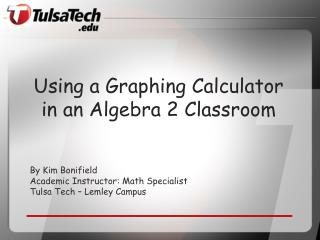# Using a Graphing Calculator in an Algebra 2 Classroom - PowerPoint PPT PresentationDownload PresentationUsing a Graphing Calculator in an Algebra 2 Classroom

Using a Graphing Calculator in an Algebra 2 ClassroomDownload Presentation## Using a Graphing Calculator in an Algebra 2 Classroom

- - - - - - - - - - - - - - - - - - - - - - - - - - - E N D - - - - - - - - - - - - - - - - - - - - - - - - - - -
##### Presentation Transcript

1. Using a Graphing Calculator in an Algebra 2 Classroom By Kim Bonifield Academic Instructor: Math Specialist Tulsa Tech – Lemley Campus

2. Why we need to teach these skills Build confidence with technology and math Calculators are a tool for learning mathematics Teaching math through discovery Skills needed in other courses – Common Core

3. The Four Calculator Skills Required on the AP Calculus Exam Graph a function in an appropriate window. Solve equations numerically, use Solver, find intersections, and graph functions. Find the numerical value of a derivative at a point. Evaluate a definite integral.

4. Philosophy Teach the math concepts using the calculator Always connect calculator skills to the math* Allow time for them to play and practice (project) Make it fun Don’t be afraid that you don’t know how to do something. Ask them. Discover together. Have students communicate what they are learning to their fellow students.

5. Tips for introductory Put a calculator in their hands as early as Algebra 1 Simple math – learn the keyboard, On button Instruct then practice, or use guided activities, or group work Practice, practice

6. Sample Algebra 2 Topics • Linear functions • Window (no zoom) • Finding intersections • Quadratic Functions • Finding zeroes, maximum/minimum • Factoring • Transformations • Applications • Matrices • Multiplication • Solving Systems of Equations (RREF)

7. Sample assignments and projects Practice Sheet – Calculations Window Calculator Exercise TI – 84+ Caching Games Mathbits.com Make These Designs Project Transformation Project

8. Resources online Websites: www.mathbits.com, YouTube videos Apps – IPad graphing calculators Quick Reference Sheets TI – Classroom Activities TI – Classroom Projects Calculator functions also used in Calculus

9. Tools to help facilitate TISmartview Emulator Software– very helpful even without a smart board Class sets of calculators TI Posters

10. Contact Kim Bonifield kim.bonifield@tulsatech.edu Email me and I will send you a copy of my PowerPoint and handouts.